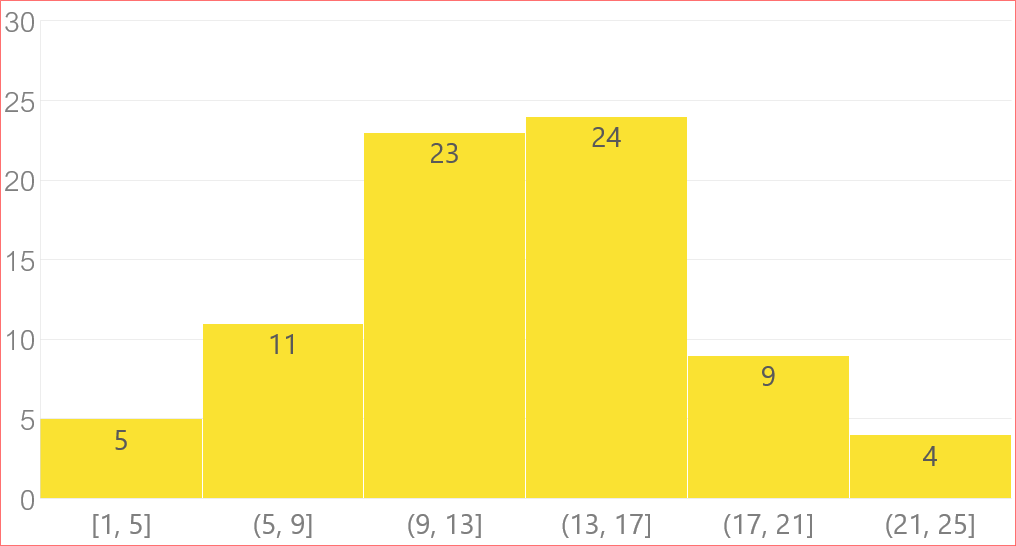This topic describes costs and cost-related concepts.

Overview

Physical optimization refers to cost-based query optimization. The costs consist of the I/O cost and the CPU cost.

Statistics

• High frequency values

High frequency values refer to common values that account for a large proportion of the total number of values. For example, in table t1, the values of field a range from 1 to 100, among which values from 1 to 10 account for 95% of the total number of values. Therefore, values from 1 to 10 are high frequency values. High frequency values are used for equality queries. The probabilities of high frequency values are evaluated.• Histogram

The histogram shows the distribution of values. For example, in table t1, the values of field a range from 1 to 100 and are divided into four groups. A total of 30 values range from 1 to 25, 20 values range from 26 to 50, 25 values range from 51 to 75, and 25 values range from 76 to 100.• Correlation coefficient

A correlation coefficient indicates the correlation between the physical sequence and logical sequence of values in a column. A high correlation indicates that the cost of scanning discrete blocks by using index scans is low.• Other statistics
• Number of unique values
• Proportion of null values
• The number of rows in a table
• The number of pages in a table

Selectivity

• Unconditional query.
EXPLAIN SELECT * FROM tenk1;

QUERY PLAN
-------------------------------------------------------------
Seq Scan on tenk1  (cost=0.00..458.00 rows=10000 width=244)

SELECT relpages, reltuples FROM pg_class WHERE relname = 'tenk1';

relpages | reltuples
----------+-----------
358 |     10000
• Range query.
EXPLAIN SELECT * FROM tenk1 WHERE unique1 < 1000;

QUERY PLAN
--------------------------------------------------------------------------------
Bitmap Heap Scan on tenk1  (cost=24.06..394.64 rows=1007 width=244)
Recheck Cond: (unique1 < 1000)
->  Bitmap Index Scan on tenk1_unique1  (cost=0.00..23.80 rows=1007 width=0)
Index Cond: (unique1 < 1000)
• Range query statement.
SELECT histogram_bounds FROM pg_stats
WHERE tablename='tenk1' AND attname='unique1';

histogram_bounds
------------------------------------------------------
{0,993,1997,3050,4040,5036,5957,7057,8029,9016,9995}

selectivity = (1 + (1000 - bucket.min)/(bucket.max - bucket.min))/num_buckets
= (1 + (1000 - 993)/(1997 - 993))/10
= 0.100697

rows = rel_cardinality * selectivity
= 10000 * 0.100697
= 1007  (rounding off)
• Equality query.
EXPLAIN SELECT * FROM tenk1 WHERE stringu1 = 'CRAAAA';

QUERY PLAN
----------------------------------------------------------
Seq Scan on tenk1  (cost=0.00..483.00 rows=30 width=244)
Filter: (stringu1 = 'CRAAAA'::name)
• Equality query statement.
SELECT null_frac, n_distinct, most_common_vals, most_common_freqs FROM pg_stats
WHERE tablename='tenk1' AND attname='stringu1';

null_frac         | 0
n_distinct        | 676
most_common_vals|{EJAAAA,BBAAAA,CRAAAA,FCAAAA,FEAAAA,GSAAAA,JOAAAA,MCAAAA,NAAAAA,WGAAAA}
most_common_freqs | {0.00333333,0.003,0.003,0.003,0.003,0.003,0.003,0.003,0.003,0.003}

selectivity = mcf  = 0.003

rows = 10000 * 0.003 = 30

## Note: If the value is not in most_common_vals, use the following formula: selectivity = (1 - sum(mvf))/(num_distinct - num_mcv)